Processing ......FreeComputerBooks.com Links to Free Computer, Mathematics, Technical Books all over the World

Applied and Computational Linear Algebra: A First Course
GIS Visualizer - Geographic Data Visualized on 40+ Maps! Click here for details.
• Title Applied and Computational Linear Algebra: A First Course
• Author(s) Charles L. Byrne
• Publisher: Chapman and Hall/CRC; 1 edition (June 23, 2000)
• Hardcover/Paperback N/A
• eBook PDF, 504 pages, 2.2 MB
• Language: English
• ISBN-10: 1584880643
• ISBN-13: 978-1584880646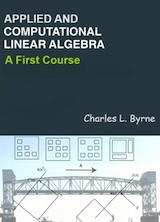Book Description

This book is intended as a text for a graduate course that focuses on applications of linear algebra and on the algorithms used to solve the problems that arise in those applications. Often the particular nature of the applications will prompt us to seek algorithms with particular properties; we then turn to the matrix theory to understand the workings of the algorithms.

• Charles L. Byrne is a Professor at University of Massachusetts, Lowell.
Reviews, Ratings, and Recommendations: Related Book Categories: Read and Download Links:Similar Books:
•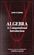Algebra: A Computational Introduction (John Scherk)

This book is a unique approach and presentation, the author demonstrates how software can be used as a problem-solving tool for algebra. It includes many computations, both as examples and as exercises.

•A Computational Introduction to Number Theory and Algebra

This introductory book emphasises algorithms and applications, such as cryptography and error correcting codes, and is accessible to a broad audience. It covers the basics of number theory, abstract algebra and discrete probability theory.

•Applied Linear Algebra: Vectors, Matrices, and Least Squares

Combines straightforward explanations with a wealth of practical examples to offer an innovative approach to teaching linear algebra. Requiring no prior knowledge of the subject, it covers the aspects of linear algebra - vectors, matrices, and least squares

•Linear Algebra (Jim Hefferon)

This textbook covers linear systems and Gauss' method, vector spaces, linear maps and matrices, determinants, and eigenvectors and eigenvalues. Each chapter has three or four discussions of additional topics and applications.

•Linear Algebra: A Course for Physicists and Engineers (Arak Mathai)

This textbook on linear algebra is written to be easy to digest by non-mathematicians. It introduces the concepts of vector spaces and mappings between them without too much theorems and proofs. Various applications of the formal theory are discussed as well.

•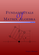Fundamentals of Matrix Algebra (Gregory Hartman)

A college (or advanced high school) level text dealing with the basic principles of matrix and linear algebra. It covers solving systems of linear equations, matrix arithmetic, the determinant, eigenvalues, and linear transformations.

•Matrix Algebra (Marco Taboga)

This is a course in matrix algebra, with a focus on concepts that are often used in probability and statistics. Matrix algebra is one of the most important areas of mathematics for data analysis and for statistical theory.

•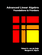Advanced Linear Algebra: Foundations to Frontiers (Robert Geijn)

The focus is on numerical linear algebra, the study of how theory, algorithms, and computer arithmetic interact. These materials keep the learner engaged by intertwining text, videos, exercises, and programming activities in consumable chunks.

•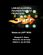Linear Algebra: Foundations to Frontiers (M. Myers, et al.)

This book is an introduction to the basic concepts of linear algebra, along with an introduction to the techniques of formal mathematics. It begins with systems of equations and matrix algebra before moving into the advanced topics.

•Computational Linear Algebra and N-dimensional Geometry

This undergraduate textbook on Linear Algebra and n-Dimensional Geometry, in a self-teaching style, is invaluable for sophomore level undergraduates in mathematics, engineering, business, and the sciences.

•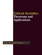Linear Algebra - Theorems and Applications (Hassan Abid Yasser)

This book contains selected topics in linear algebra, which represent the recent contributions in the most famous and widely problems. It includes a wide range of theorems and applications in different branches of linear algebra, etc.

•A First Course in Linear Algebra (Robert A Beezer)

Linear Algebra: Foundations to Frontiers (LAFF) is packed full of challenging, rewarding material that is essential for mathematicians, engineers, scientists, and anyone working with large datasets.

•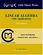Linear Algebra with Applications (W. Keith Nicholson)

The aim of the text is to achieve a balance among computational skills, theory, and applications of linear algebra. It is a relatively advanced introduction to the ideas and techniques of linear algebra targeted for science and engineering students.

Book Categories
 :All CategoriesTop Free BooksRecent BooksMiscellaneous BooksComputer EngineeringComputer LanguagesComputer ScienceData Science/DatabasesJava and Java EE (J2EE)Linux and UnixMathematicsMicrosoft and .NETMobile ComputingNetworking and CommunicationsSoftware EngineeringSpecial TopicsWeb Programming
Other Categories